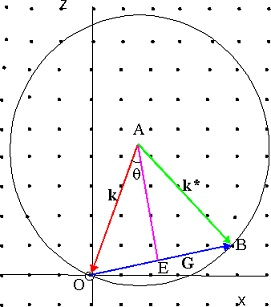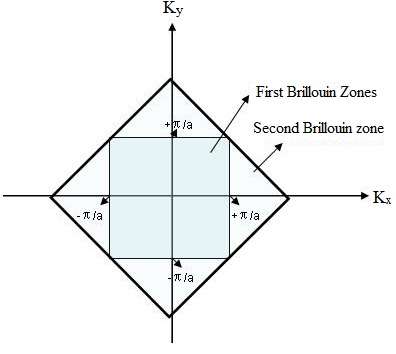#### Crystal diffraction-II, Physics tutorial

Introduction:

To describe the theory of X-ray diffraction through crystal planes, Ewald proposed the concept of reciprocal lattice. According to this theory, the illustration of interpenetrating planes within a crystal could be obtained in space via means of a set of points. Therefore the properties of planes and points are interchangeable. The space made from such points is termed as the reciprocal lattice.

Definition:

The reciprocal space lattice is the set of imaginary points constructed in such a manner that the direction of the vector from one point to the other coincides by the direction of a normal to the real space planes and the separation of such points (that is, absolute value of the vector) is equivalent to the reciprocal of the real inter planar distance.

Reciprocal Lattice:

For an ideal single crystal, the reciprocal lattice is an infinite periodic 3-D (three-dimensional) array of points whose spacing is inversely proportional to the distances between the planes in the direct lattice. The axis vector of the reciprocal lattice is represented by the equation:

A = 2π (bxc/a.bxc)

B = 2π (cxa/a.bxc)

C = 2π (axb/a.bxc)

If a, b and c are the primitive vectors of crystal lattice, then A, B, C are primitive vectors of the reciprocal lattice. Each and every vector is orthogonal to two of the axis vectors of the crystal lattice. Therefore A, B, C has property:

A.a = 2π       B.a = 0       C.a = 0

A.b = 0        B.b = 2π      C.b = 0

A.c = 0        B.c = 0        C.c = 2π

Any random set of primitive vectors a, b, c of a particular crystal lattice leads to the similar set of reciprocal lattice points.

G = hA + kB + lC (Here, h, k, l are integers)

Any vector G of the form in the above equation is termed as a reciprocal lattice vector. Each and every crystal structure consists of two lattices related with it, the crystal lattice and the reciprocal lattice. A diffraction pattern of a crystal is a map of the reciprocal lattice of the crystal; a microscopic image, if it could be solved on a fine scale, symbolizes a map of the crystal structure in real space. Whenever we rotate a lattice crystal, we rotate both the direct lattice and the reciprocal lattice. The vectors in crystal lattice encompass the dimensions of [length]; vectors in the reciprocal lattice have the dimensions of [length]-1. In dealing with wave properties of the crystals, it is suitable to define the reciprocal lattice vector G as:

G = 2π (hA + kB + lC)

This in conjunction by the first equation yields:

G.Rn = 2π (hn1 + kn2 + ln3) = 2π x integer

Therefore each and every vector of the equation (G = hA + kB + lC) satisfies the condition:

exp [iG . Rn] = 1

A few of the elementary properties of the reciprocal lattice are as:

1) The unit cell of the reciprocal lattice require not be a parallelepiped.

2) Simple cubic lattice is its own reciprocal, therefore is the hcp. On the other hand, bcc and fcc are reciprocal of one other.

3) The volume of a unit cell of the reciprocal lattice is inversely proportional to the volume of the unit cell of the direct lattice.

4) If A is the matrix of the components of A1, B1, C1 and B for those of A2, B2, C2 then B = A-1

The properties of the reciprocal lattice which make it of significance in the diffraction theory are:

1) The vector G (hkl) from the origin to the point (h, k, l) of reciprocal lattice is normal to the (hkl) plane of the crystal lattice.

2) The length of the vector G (hkl) is equivalent to the reciprocal of the spacing of the planes (hkl) of the crystal lattice.

Ewald's Construction in the reciprocal lattice:

The X-ray diffraction experiment is taken out in such a way that the wavelength and the direction for the incident X-ray beam are acknowledged. This information might be put into the reciprocal lattice as shown in the figure below.1) Select a point according to the orientation of the specimen with respect to the incident beam.

2) Draw a vector 'AO' in the incident direction of length 2π/λ terminating at the origin.

3) Draw a circle of radius 2π/λ having center at A. Note whether this circle passes via any point of the reciprocal lattice; if it does:

4) Draw a vector 'AB' to the point of the intersection.

5) Draw a vector 'OB' to the point of the intersection.

6) Draw a line 'AE' perpendicular to the 'OB'.

7) Complete the construction to all the intersection points in the similar way.

We have the given facts:

1) As OB ends on a point in the reciprocal lattice, it is normal to several set of lattice planes and is of length 2π/d, the interplanar spacing for the set. By statement, OB = 2π/d.

2) As AO = 2π/λ, then OE = 2π/λ sinθ and OB = 2.(2π/λ) sinθ.

3) As OB is normal to a lattice plane, AE is the lattice plane, and θ is the angle between the incident wave AO and the lattice plane. This is equivalent to the angle between the lattice plane AE and reflected wave AB.

4) As 2π/d = 2.(2π/λ) sinθ, we get λ = 2d sinθ; therefore the Bragg law is satisfied.

As a result, knowing the direction and wavelength of the incident wave, we have been capable to find out which plane will diffract it.

Bragg Law in Vector Form:

By means of the Ewald construction we can represent the Bragg law in vector form: Let G = OB and k = AO. For diffraction, it is essential that the vector k + G, that is, the vector AB, be equivalent in magnitude to the vector k or

(k + G)2 = k2

Or 2k. G + G2 = 0       (i)

If we call the scattered wave vector k*, than

k* = k + G       (ii)

Therefore we can write:

k*2 = k2           (iii)

And k* - k = G, representing (ii) that the scattering changes only the direction on k, and (iii) that the scattered wave distinct from the incident wave through a reciprocal lattice vector G.

Equations (ii) and (iii) are the momentum and energy conservation law for the x-ray diffraction that is an illustration of the elastic scattering.

Whether or not the k circle intersects a lattice vector, and therefore reflects, based on its magnitude and orientation. By using (i) we can construct in the reciprocal lattice the locus of all such waves which can produce Bragg reflection. This locus symbolizes a set of planes in three dimensions. The volume terminated by such planes is termed as Brillouin zone.

Brillouin Zones:

For solid state physics, the most significant statement of the diffraction condition was represented by Brillouin. The figure above represents that incident wave and reflected wave form an equivalent angle by the lattice plane AE, which is, thus, a reflecting plane. The reciprocal lattice vector G = OB is perpendicular to the reflecting plane AE. Therefore, corresponding to G = OB, the reflecting plane is AE (generated). From the relation k′ = k + G, we observe that (AO + OE) OB = 0. That is AE. OB = 0. Therefore, AE is perpendicular to OB and as well bisects it, as 'E' is the midpoint of OB by construction. Therefore, for a given reciprocal lattice vector, its right bisector is the reflecting plane. One can expand the method for determining the reflecting planes corresponding to reciprocal lattice vectors joining the reciprocal lattice point O (origin) by its neighbors in reciprocal space. The volume bounded by such planes is termed to as the geometrical statement of the first Brillouin zone (BZ).Mathematically the reflecting planes and therefore the Brillouin zones could be computed. For the simple square lattice (of lattice constant a), the reciprocal lattice vectors are:

G = 2π/a (n1i + n2j)

The wave vector for an X-ray computed from the origin of the reciprocal lattice is as:

k = kxi + kyj

Using the above two equation, we get:

n1kx + n2ky = (n12 + n22) (π/a)

By assigning various values to n1, n2, we can get different reflection lines. Therefore all k-vectors originating at the origin and ending on such lines will generate Bragg reflection.

Tutorsglobe: A way to secure high grade in your curriculum (Online Tutoring)